# Force Magnitude Tension Elastic Force Gravity Normal Force

• Slides: 14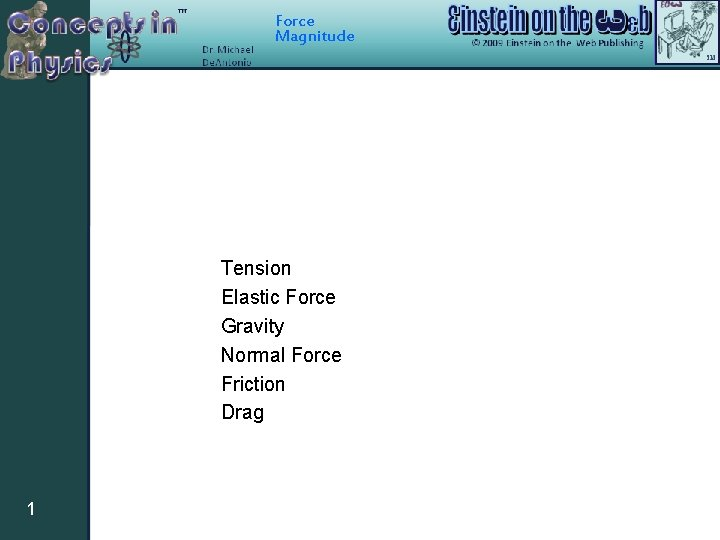Force Magnitude Tension Elastic Force Gravity Normal Force Friction Drag 1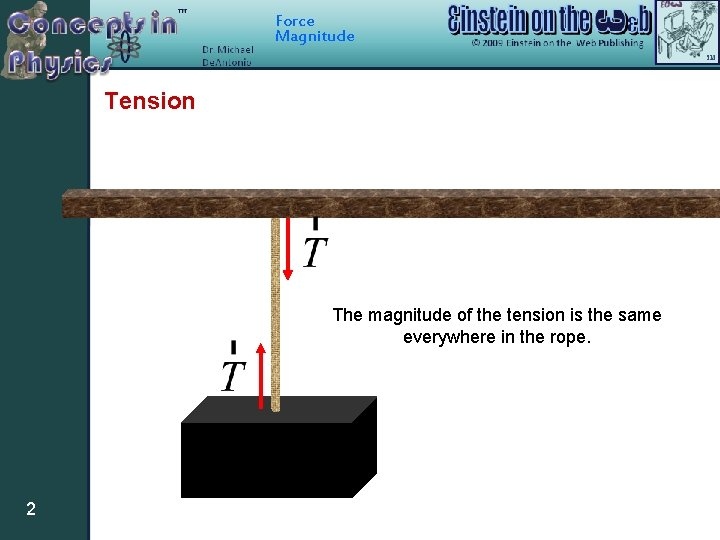Force Magnitude Tension The magnitude of the tension is the same everywhere in the rope. 2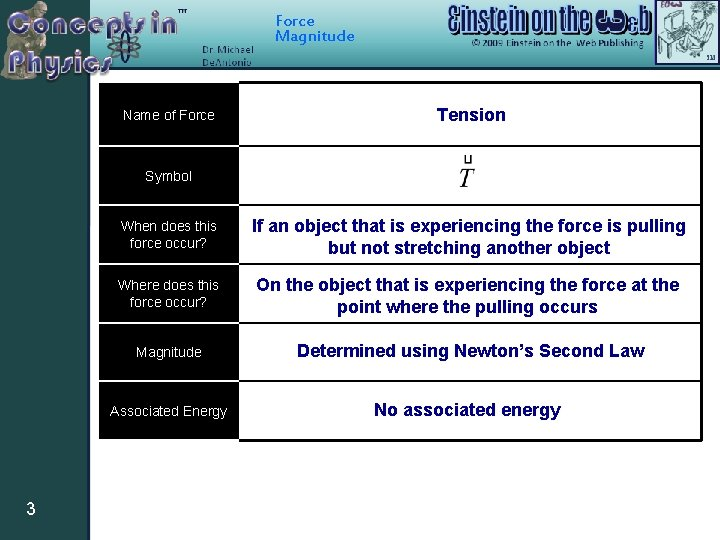Force Magnitude Name of Force Tension Symbol 3 When does this force occur? If an object that is experiencing the force is pulling but not stretching another object Where does this force occur? On the object that is experiencing the force at the point where the pulling occurs Magnitude Determined using Newton’s Second Law Associated Energy No associated energy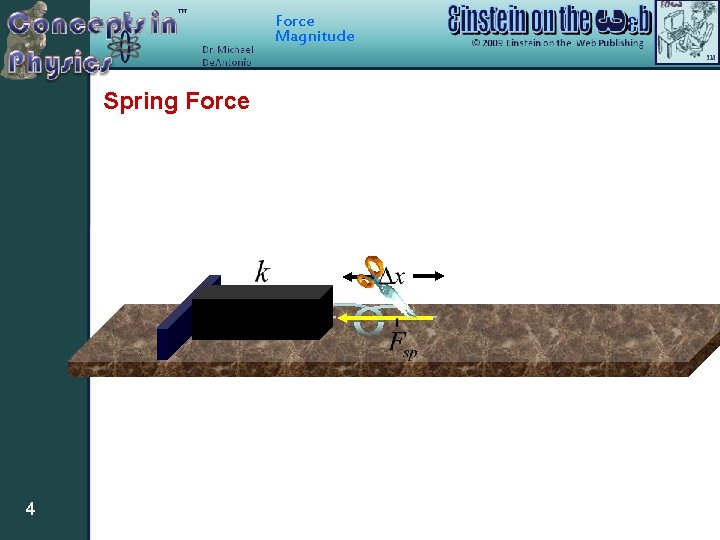Force Magnitude Spring Force 4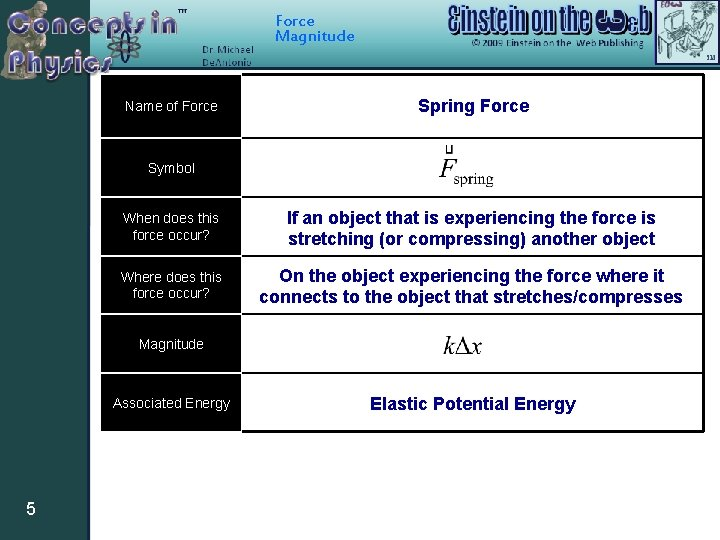Force Magnitude Name of Force Spring Force Symbol When does this force occur? If an object that is experiencing the force is stretching (or compressing) another object Where does this force occur? On the object experiencing the force where it connects to the object that stretches/compresses Magnitude Associated Energy 5 Elastic Potential EnergyForce Magnitude Gravity 6Force Magnitude Name of Force Gravity Symbol or When does this force occur? If there are two massive objects present Where does this force occur? At the center-of-mass of the object receiving the force Magnitude Associated Energy 7 or Gravitational Potential Energy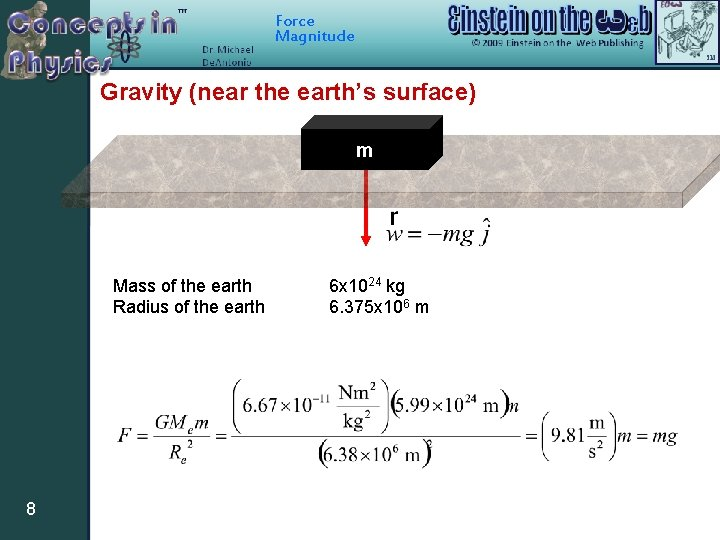Force Magnitude Gravity (near the earth’s surface) m Mass of the earth Radius of the earth 8 6 x 1024 kg 6. 375 x 106 m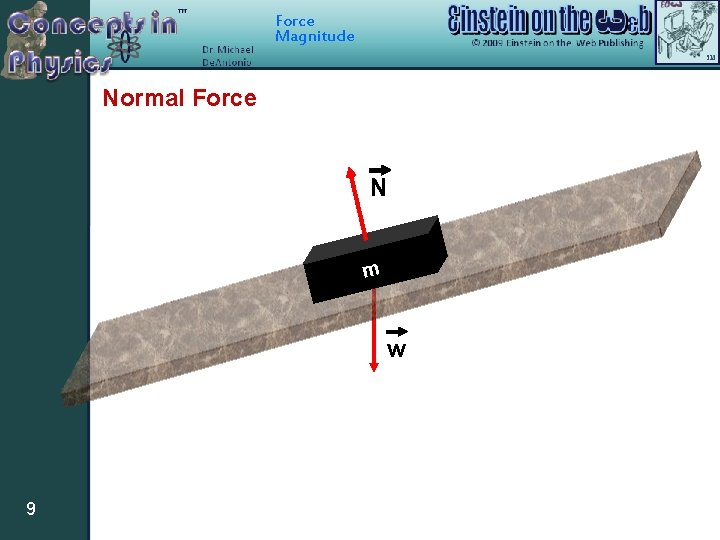Force Magnitude Normal Force N m w 9Force Magnitude 10 Name of Force Normal Force Symbol or When does this force occur? If an object touches the surface of another object Where does this force occur? On the object receiving the force at the point(s) where it touches the surface Magnitude Determined using Newton’s Second Law Associated Energy No associated energyForce Magnitude Friction N n or o i t mo f o n o i t ion c Dire ble mot i poss 11 f mForce Magnitude 12 Name of Force Friction Symbol or When does this force occur? If an object touches the surface of another object Where does this force occur? On the object receiving the force at the point(s) where it touches the surface Magnitude or Associated Energy Thermal Energy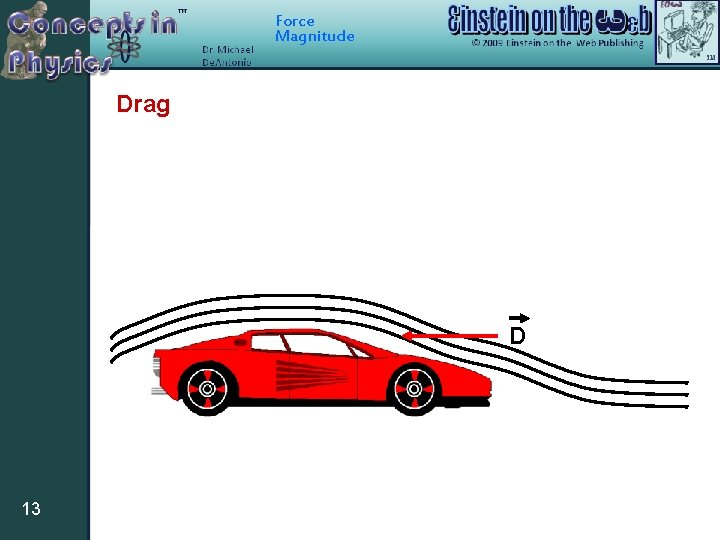Force Magnitude Drag D 13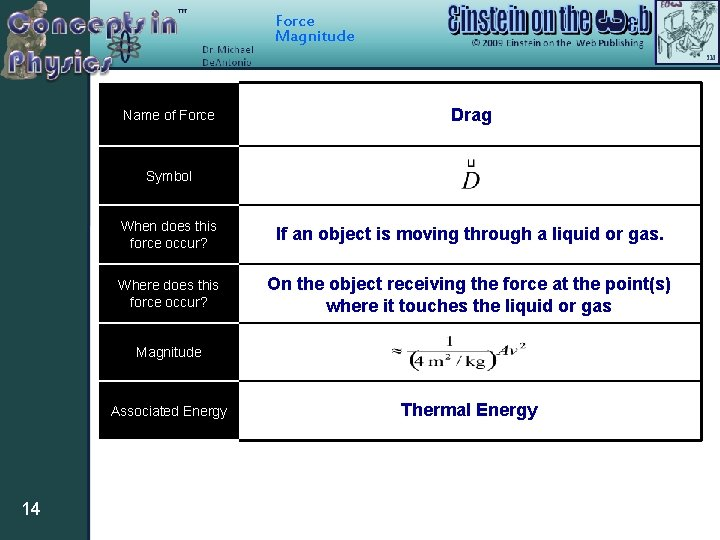Force Magnitude Name of Force Drag Symbol When does this force occur? If an object is moving through a liquid or gas. Where does this force occur? On the object receiving the force at the point(s) where it touches the liquid or gas Magnitude Associated Energy 14 Thermal Energy